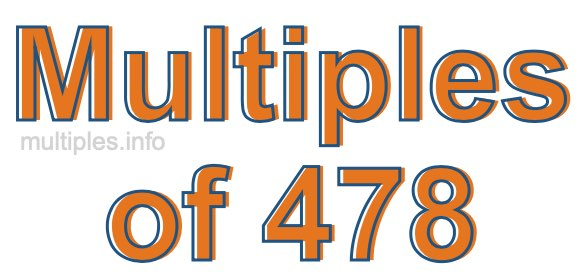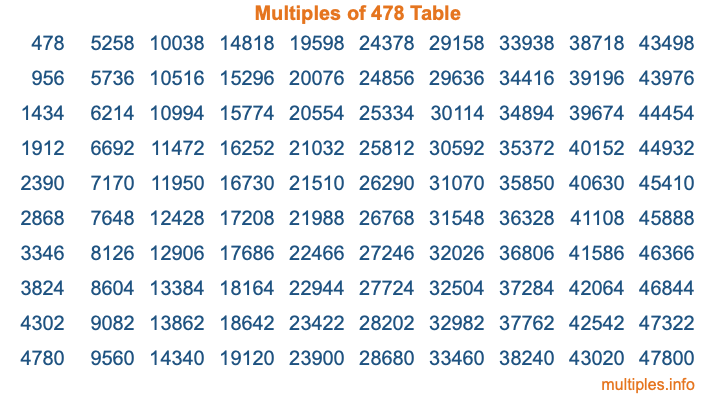Multiples of 478Welcome to the Multiples of 478 page. Here we will first teach you everything you will ever need to know about the multiples of 478, and then give you a study guide summary of everything we taught you to make sure you remember it all. Use this page to look up facts and learn information about the multiples of 478. This page will make you a multiples of four hundred seventy-eight expert!

Definition of Multiples of 478
Multiples of 478 are all the numbers that when divided by 478 equal an integer. Each of the multiples of 478 are called a multiple. A multiple of 478 is created by multiplying 478 by an integer.

Therefore, to create a list of multiples of 478, you start with 1 multiplied by 478, then 2 multiplied by 478, then 3 multiplied by 478, and so on for as long as you want. Thus, the list of the first five multiples of 478 is 478, 956, 1434, 1912, and 2390. To see a larger list of multiples of 478, see the printable image of Multiples of 478 further down on this page. We also have a category where you can choose any nth multiple of 478.

Multiples of 478 Checker
The Multiples of 478 Checker below checks to see if any number of your choice is a multiple of 478. In other words, it checks to see if there is any number (integer) that when multiplied by 478 will equal your number. To do that, we divide your number by 478. If the the quotient is an integer, then your number is a multiple of 478.

Is  a multiple of 478?

Least Common Multiple of 478 and ...
A Least Common Multiple (LCM) is the lowest multiple that two or more numbers have in common. This is also called the smallest common multiple or lowest common multiple and is useful to know when you are adding our subtracting fractions. Enter one or more numbers below (478 is already entered) to find the LCM.

Check out our LCM Calculator if you need more details about the Least Common Multiple or if you need the LCM for different numbers for adding and subtraction fractions.

nth Multiple of 478
As we stated above, 478 is the first multiple of 478, 956 is the second multiple of 478, 1434 is the third multiple of 478, and so on. Enter a number below to find the nth multiple of 478.

th multiple of 478

Multiples of 478 vs Factors of 478
478 is a multiple of 478 and a factor of 478, but that is where the similarities end. All postive multiples of 478 are 478 or greater than 478. All positive factors of 478 are 478 or less than 478.

Below is the beginning list of multiples of 478 and the factors of 478 so you can compare:

Multiples of 478: 478, 956, 1434, 1912, 2390, etc.

Factors of 478: 1, 2, 239, 478

As you can see, the multiples of 478 are all the numbers that you can divide by 478 to get a whole number. The factors of 478, on the other hand, are all the whole numbers that you can multiply by another whole number to get 478.

It's also interesting to note that if a number (x) is a factor of 478, then 478 will also be a multiple of that number (x).

Multiples of 478 vs Divisors of 478
The divisors of 478 are all the integers that 478 can be divided by evenly. Below is a list of the divisors of 478.

Divisors of 478: 1, 2, 239, 478

The interesting thing to note here is that if you take any multiple of 478 and divide it by a divisor of 478, you will see that the quotient is an integer.

Multiples of 478 Table
Below is an image of the first 100 multiples of 478 in a table. The table is in chronological order, column by column. The first column has the first ten multiples of 478, the second column has the next ten multiples of 478, and so on.The Multiples of 478 Table is also referred to as the 478 Times Table or Times Table of 478. You are welcome to print out our table for your studies.

Negative Multiples of 478
Although not often discussed or needed in math, it is worth mentioning that you can make a list of negative multiples of 478 by multiplying 478 by -1, then by -2, then by -3, and so on, to get the following list of negative multiples of 478:

-478, -956, -1434, -1912, -2390, etc.

Multiples of 478 Summary
Below is a summary of important Multiples of 478 facts that we have discussed on this page. To retain the knowledge on this page, we recommend that you read through the summary and explain to yourself or a study partner why they hold true.

There are an infinite number of multiples of 478.

A multiple of 478 divided by 478 will equal a whole number.

478 divided by a factor of 478 equals a divisor of 478.

The nth multiple of 478 is n times 478.

The largest factor of 478 is equal to the first positive multiple of 478.

478 is a multiple of every factor of 478.

478 is a multiple of 478.

A multiple of 478 divided by a divisor of 478 equals an integer.

478 divided by a divisor of 478 equals a factor of 478.

Any integer times 478 will equal a multiple of 478.

Multiples of a Number
Here you can get the multiples of another number, all with the same attention to detail as we did for multiples of 478 on this page.

Multiples of
Multiples of 479
Did you find our page about multiples of four hundred seventy-eight educational? Do you want more knowledge? Check out the multiples of the next number on our list!